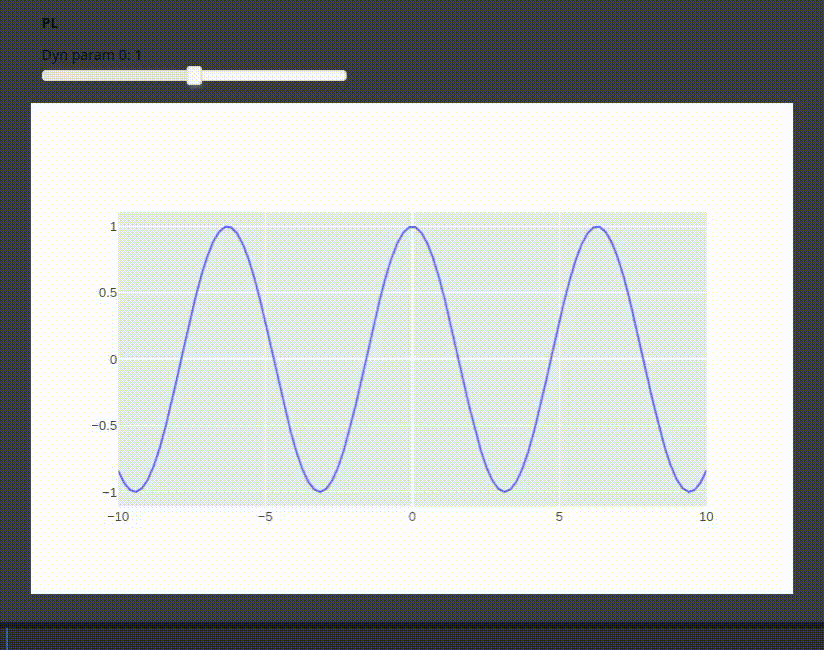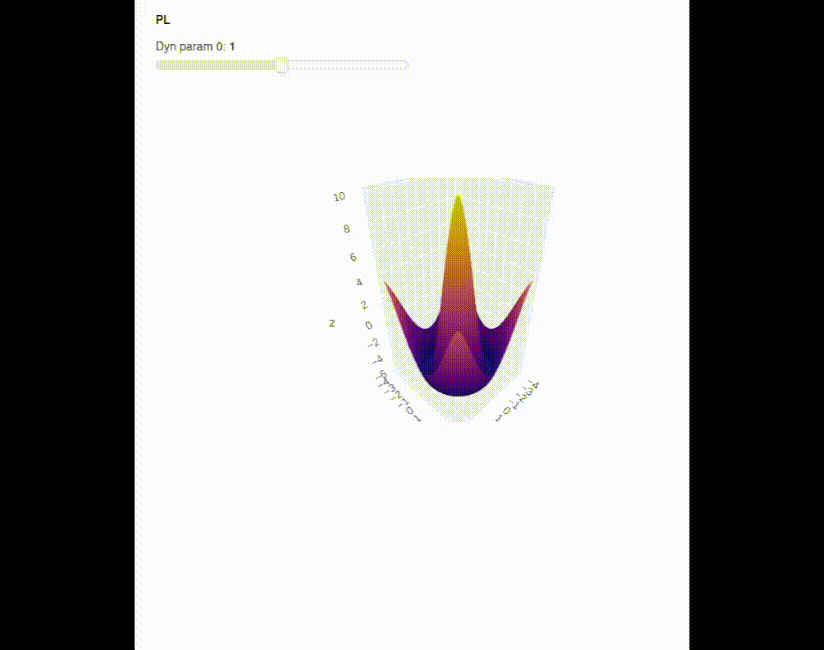# Plotly pane resizes after a while

As I’m trying to understand how to use `pn.pane.Plotly` I have experienced a couple of issues. They are probably related to my inexperience, so I’m asking for help.

#### First example:

After running the application, it might happen that Plotly pane increases its height. This is annoying because:

• if the app is run inside Jupyter Notebook, the resizing prevents the the x-axis to be visible. Users have to scroll down.
• if the app is served to a new browser window, the pane is going to use all the available height.
``````import param
import panel as pn
pn.extension("plotly")
import numpy as np
import plotly.graph_objects as go

class PL(param.Parameterized):
check_val = param.Integer(default=0)

def __init__(self, func, *parameters):
super().__init__()

# remove the previous class attributes added by the previous instances
cls_name = type(self).__name__
setattr(type(self), "_" + cls_name + "__params", dict())
prev_params = [k for k in type(self).__dict__.keys() if "dyn_param_" in k]
for p in prev_params:
delattr(type(self), p)

self.mapping = {}
# create and attach the params to the class
for i, p in enumerate(parameters):
pname = "dyn_param_{}".format(i)
# TODO: using a private method: not the smartest thing to do
self.param.watch(self._increment_val, pname)
self.mapping[i] = pname

self.func = func
self.x = np.linspace(-10, 10, 100)
y = func(self.x, 1)
self.fig = go.Figure([
go.Scatter(x=self.x, y=y)
])
self.pane = pn.pane.Plotly(self.fig)

def _increment_val(self, *depends):
self.check_val += 1

for k, v in self.mapping.items():

@param.depends("check_val", watch=True)
def _update(self):
self.fig.data["x"] = self.x
self.fig.data["y"] = y
self.pane.object = self.fig

def show(self):
return pn.Column(pn.Param(self, parameters=list(self.mapping.values())), self.pane)

f = lambda x, t: np.cos(x * t)
c = PL(f, param.Number(default=1, softbounds=(0, 2)))
c.show()
``````It is interesting to note that if I return just the Plotly figure, everything works fine with no resizing.

``````    def show(self):
return pn.Column(pn.Param(self, parameters=list(self.mapping.values())), self.fig)
``````

#### Second example

On top of the resizing problem mentioned earlier, this example exhibits a new problem only when the application is served to a browser window. In particular, it seems like the application freezes and keeps repeating a few updates over and over again, even when the click has been released from the slider.

``````import param
import panel as pn
pn.extension("plotly")
import numpy as np
import plotly.graph_objects as go

class PL(param.Parameterized):
check_val = param.Integer(default=0)

def __init__(self, func, *parameters):
super().__init__()

# remove the previous class attributes added by the previous instances
cls_name = type(self).__name__
setattr(type(self), "_" + cls_name + "__params", dict())
prev_params = [k for k in type(self).__dict__.keys() if "dyn_param_" in k]
for p in prev_params:
delattr(type(self), p)

self.mapping = {}
# create and attach the params to the class
for i, p in enumerate(parameters):
pname = "dyn_param_{}".format(i)
# TODO: using a private method: not the smartest thing to do
self.param.watch(self._increment_val, pname)
self.mapping[i] = pname

self.func = func
x = y = np.linspace(-4, 4, 50)
self.x, self.y = np.meshgrid(x, y)
z = func(self.x, self.y, 0.15)
self.fig = go.Figure([
go.Surface(x=self.x, y=self.y, z=z)
])
self.pane = pn.pane.Plotly(self.fig)

def _increment_val(self, *depends):
self.check_val += 1

for k, v in self.mapping.items():

@param.depends("check_val", watch=True)
def _update(self):